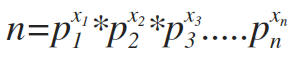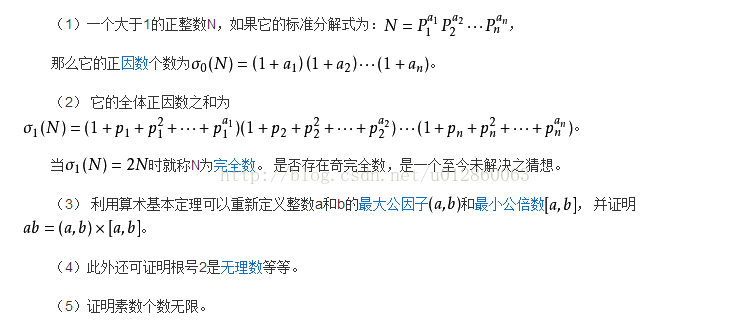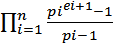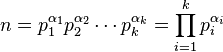• 这里讲一下算法中常用到的唯一因子分解定理： 合数a仅能以一种方式写成如下乘积形式： a = p1^e1*p2^e2*...*pr^er 其中pi为素数，p1<p2<...<pr，且ei为正整数。例如数6000=2^4*3*5^3。 证明就不讲了，...
这里讲一下算法中常用到的唯一因子分解定理：
合数a仅能以一种方式写成如下乘积形式：
a = p1^e1*p2^e2*...*pr^er     其中pi为素数，p1<p2<...<pr，且ei为正整数。例如数6000=2^4*3*5^3。
证明就不讲了，网上一抓一大把，这里应用这个定理写了一个类，可以在分解合数的时候增加效率。
#include <iostream>
#include <vector>
#include <cstring>

using namespace std;

#define maxn 65535

class Resolve
{
public:
bool list[maxn];
vector<long long> v;
Resolve()
{
find_Prime();
}
void find_Prime()
{
memset(list,true,sizeof(list));
for(long long i=2;i<=maxn;i++)
{
for(long long j=i*i;j<=maxn;j+=i)
{
list[j]=false;
}
}
for(long long i=2;i<=maxn;i++)
{
if(list[i])
v.push_back(i);
}
}
vector<long long> reso(long long x)
{
vector<long long>ans;
long long k=0;
while(x>1)
{
if(x%v[k]==0)
{
ans.push_back(v[k]);
x/=v[k];
continue;
}
k++;
}
return ans;
}
~Resolve()
{
vector<long long>().swap(v);
delete[] list;
}
};
最后再附上释放向量vector内存的方法。
//方法一
vector<int>v;
//释放语句
vector<int>().swap(v);

//方法二，动态创建对象时
vector<int> *v=new vector<int>();
//释放语句
delete[] v;

展开全文算法学习
• 1.唯一分解定理，也叫算术基本定理，指的是任何n>=2,都可以分解为n=p1* p2 * p3 * … * pn，其中pi为质数。 其包括两个断言： 断言1：数n可以以某种方式分解成素数乘积。 断言2：仅有一种这样的因数分解。（除...
1.唯一分解定理，也叫算术基本定理，指的是任何n>=2,都可以分解为n=p1* p2 * p3 * … * pn，其中pi为质数。
其包括两个断言：
断言1：数n可以以某种方式分解成素数乘积。
断言2：仅有一种这样的因数分解。（除因数重排外）。
其可以化简为另有一些推论：推论（2）中的公式还可以进一步推导为写法一，对单个n的时间复杂度为O(n)： 求n的所有素因子数列a
public static void main(String args[])
long n=0;
int maxn=100007;//存起来绰绰有余,毕竟2的100次方就有18位了
long a[]=new long[maxn];
int cnt=0;
for(long i=2;i*i<=n;i++) {
while(n%i==0) {
a[cnt++]=i;
n/=i;
}
}
if(n!=1) {//如果n是一个合数，那么它的分解素因子只可能有一个大于sqrt(n)的；
//原因：设d能整除n,则n=d*n/d,则min(d,n/d)<=sqrt(n)。
a[cnt++]=n;
}
}

写法二，先把一定范围内的素数筛出来，然后再让n对素数进行遍历除等。对大量n比写法一更省时间。
展开全文• 唯一分解定理是数论中重要的思想之一。简述就是一个大于一的整数N,可以由他的素因子唯一构成。证明用到了反证法。 数学 假设N是大于一的正整数，我们有： N=q1^p1*q2^p2*……*qn^pn q1……qn是N的质因数 有...
简介
唯一分解定理是数论中重要的思想之一。简述就是一个大于一的整数N,可以由他的素因子唯一构成。证明用到了反证法。
数学
假设N是大于一的正整数，我们有：
N=q1^p1*q2^p2*……*qn^pn

q1……qn是N的质因数 有如下性质：
 1. 约数个数 = (p1+1)*(p2+1)*……*(pn+1)
2. 约数和 = (1+q1+……+q1^p1)*(1+q2+……+q2^p2)*……*(1+qn+……+qn^pn)

质因数分解
for(int i=2;i*i<=n;i++)
{
if(n%i==0)
{
a[k++]=i;//将素因数存在数组中
while(n%i==0)n/=i;//将所有的素因数除掉
}
if(n==1)break;
}
if(n!=1)a[k++]=n;

展开全文• 算术基本定理（唯一分解定理） 一句话：  　任何大于１的自然数，都可以唯一分解成有限个质数的乘积 例如对于大于1的自然数n，  这里Pii均为质数，其指数aii是正整数。  这样的分解称为的标准分解式。 唯一...
算术基本定理（唯一分解定理）
一句话：  　　　　 任何大于１的自然数，都可以唯一分解成有限个质数的乘积
例如对于大于1的自然数n，这里Pii均为质数，其指数aii是正整数。  这样的分解称为的标准分解式。

唯一分解定理具有：  　①唯一性（分配方式的唯一性）  　②存在性

证明：
百度百科+自己胡搞了+自己以前做的笔记
①唯一性  　　首先明确一个事实，若p是ab的约数（p|ab,p可以整除ab），则p不是a的约数，就是b的约数。  　　如果p是a的约数则证毕。如果p不是a的约数，则p和a的最大公约数为1。  　　则由裴蜀定理推得，因为使a,b互质的充要条件是存在整数x,y使ax+by=1。  　　于是b=b(ma+np) =abm+bnp（……）；  　　因为先前已经知道p是ab的约数，则上式右边两项都可以被p整除。  　　所以p就是b的约数。  　　唯一性得证。  　　  ②存在性  　　假设n为不能被分为质数的乘积的自然数之一，切n为最小  　　因为设n为大于1的合数（如果n为质数，则只有n=n，显然这是质数的乘积）  　　因为每个合数都可以分为两个大于1小于它的两自然数的乘积  　　所以n=a×b  　　又因为n为不能被分为质数的乘积的自然数中最小的一个  　　所以a和b可以分为质数的乘积  　　所以n已就可以分为质数的乘积，与假设不符合，故假设错误  　　存在性得证。

定理应用：
（1）一个大于1的正整数N，如果它的标准分解式为：，那么它的正因数个数为（2） 它的全体正因数之和为当时就称N为完全数。 是否存在奇完全数，是一个至今未解决之猜想。
（3） 利用算术基本定理可以重新定义整数a和b的最大公因子 gcd(a,b)
和最小公倍数 lcm(a,b), 并证明
a*b=gcd(a,b) * lcm(a,b)
(原来这里直接用的结论，现在知道如何证明的了，证明见下)
（4）此外还可证明根号2是无理数等等。
（5）证明素数个数无限。

证明（3）：（用唯一分解定理）
现在我们来看下下下面这个式子：  　　已知gcd[最小公约数] (a,b)，lcm[最大公倍数] (a,b)；  　　a×b=gcd(a,b)×lcm(a,b)  　　  　　a=12;b=14  　　gcd(a,b)=2 ; lcm(a,b)=84 ;  　　tot=168 [gcd(a,b)×lcm(a,b)]  　　a×b＝12×14=168  　　然后  　　12=3×4  　　14=2×7  　　：  　　：  　　12=2^1×2^1×3^1  　　14=2^1×7^1  　　所以 max=7^1×3^1×=21  　　　　 min=2^1×2^1×2^1=8  　　　　 min×max＝168 = gcd(a,b)×lcm(a,b) = a×b  　　  　　所以gcd(a,b)×lcm(a,b) = a×b  　　  　　证明：  　　设x=gcd(a,b)，y=lcm(a,b)  　　则a=m×x，b=n×x,m与n互质  　　故y=m×n*x  　　因此x×y=x×(m×n×x)=(m×x)×(n×x)=a×b  　　即a×b=gcd(a,b)×lcm(a,b)
证明完毕

另加一个用欧拉函数求小于等于n的和n互质的数的数量  公式：
phi(n) = n(1-1/p1)(1-1/p2)……(1-1/pn)      容斥很爆炸
例如 n=12
12=2^2*3^1
phi(12)=12*(1-1/2)*(1-1/3)=4
与 12 互质的数量是 4个，分别是 1,5,7,11
展开全文• 唯一分解定理又称算术基本定理，指：一个大于一的正整数N都可以唯一分解成有限个质数的乘积， N=p1a1 * p2a2 * p3a3 * … * pnan，这里p1< p2< p3 <…< pn均为质数，ai均为正整数.这样的式子成为N的标准...gcd 算法导论 算法
• ## 数论-唯一分解定理

千次阅读 2015-05-04 21:34:32
唯一分解定理 唯一分解定理 最小剩余 定理 定义 推论 模 定理 证明 推论 唯一分解 定理 证明 存在 唯一 总结 参考最小剩余定理 设aa和bb为整数,b>0b>0,则存在整数qq和rr,使得a=qb+r,0≤r, 0 \le r,使rr称为bb除aa...数学
• 分解因子 Time Limit: 1500ms Memory limit: 10000K 有疑问？点这里^_^ 题目描述 假设x是一个正整数，它的值不超过65535(即1 输入 输入的第一行含一个正整数k (1 输出 每个测试例对应一行输出，...
• 质因数分解（唯一分解定理） 基本概念： 每个合数都可以写成几个质数相乘的形式，其中每个质数都是这个合数的因数，叫做这个合数的分解质因数。 分解质因数只针对合数。 并且，每个合数能够且仅仅能够被分解为唯...质因数分解
• 唯一因数分解定理: 每一个整数n>1都可以用唯一的方法表示为素因数之积,不同之处至多只能是因数的次序. 以此为前提, 显然无最大素因数,否则将存在最大整数,而整数的归纳集无上确界最大元.以下网络的转载:不存在最大...网络
• ...唯一分解定律：又称为正整数的唯一分解定理，即：每个大于1的自然数均可写为质数的积，而且这些素因子按大小排列之后，写法仅有一种方式。 当题目有大数相除，求余数时，精度要求高时...
• 点击打开链接#include&lt;cstdio&gt; #include&lt;cmath&gt; using namespace std; int n, ans=1; int main(){ int t; scanf("%d", &amp;t); while(t--) ... ...
• 又称“自然数唯一分解定理”。任一大于1的自然数都可分解成若干质因数的连乘积，如果不计各质因数的顺序，这种分解是唯一的。 如：825＝3·52·11,100=2^2*5^2。 这样的分解称为N的标准分解式。最早证明是由...
• 算术基本定理，又称为正整数的唯一分解定理，即：每个大于1的自然数均可写为质数的积，而且这些素因子按大小排列之后，写法仅有一种方式。例如: 证明略（其实是我不懂我会乱说XD），如果需要证明就百度吧，上面有...
• 看《什么是数学》有讲一个算术基本定理，又称为正整数的唯一分解定理，即：每个大于1的自然数均可写为质数的积，而且这些素因子按大小排列之后，写法仅有一种方式。看了半天还是不太明白是怎么证明的。贴一段转来的...
• 算数基本定理（唯一分解定理） 每个大于1的自然数均可写为质数的积，而且这些素因子按大小排列之后，写法仅有一种方式。 \$ \ \ \ \ \ \ \ \ \ \ \ \ \ \ \ \ \ \ \ \ \ \ \ \ \ \ \ \ \ \ \ \ \ \ \ \ \ \ \ \ \ \ \...
• 这个唯一分解定理一般在ACM中经常用到，我在做数论的时候发现还有这么一个东西，本以为要暴力解决的事情（当然超时），现在可以有这么一个定理使用，那可是非常开心了，也就有了下面的详细介绍： 根据上面的定理可知...
• UVA10791 一个数n的唯一分解式个部分和最小 证明需要知道诶，自己百度 ...4.这种放超时的唯一分解定理分解方式要会 #include #include #include using namespace std; int main() { int n,m; unsigned ...
• 链接：https://www.nowcoder.com/acm/contest/90/F来源：牛客网题目描述给定n，求1/x + 1/y = 1/n （x&lt;=y）的解数。（x、y、n均为正整数） 输入描述:在第一行输入一个正整数T。...首先明白一个定理唯一分...蓝桥杯 算数基本定理
• 那时候，我们不会是思考为什么这种分解是存在的，更不会思考为什么这种分解唯一的。我们把老师和课本当成是权威，所说所写都是真理。稍微肯思考的孩子，动手写了几个例子，发现也确实是对的。那时候，一切只需要...
• 前几天了解了唯一分解定理，看了之后感觉定理内容挺简单的，不知道这个定理能干什么，算是比较巧吧，这几天一下遇到了两道需要用唯一分解定理的题目。 唯一分解定理内容 两道例题 ...
• 算术基本定理，又称为正整数的唯一分解定理，即：每个大于1的自然数均可写为质数的积，而且这些素因子按大小排列之后，写法仅有一种方式。例如:   算术基本定理的内容由两部分构成： 分解的存在性； 分解的唯一......